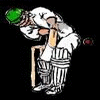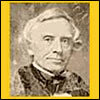#### You may also like### Tree Tops

Can you make sense of information about trees in order to maximise the profits of a forestry company?### Cricket Ratings

Like all sports rankings, the cricket ratings involve some maths. In this case, they use a mathematical technique known as exponential weighting. For those who want to know more, read on.### History of Morse

This short article gives an outline of the origins of Morse code and its inventor and how the frequency of letters is reflected in the code they were given.

# Which List Is Which?

### Why do this problem?

Students grow accustomed to thinking that calculating an average gives you all the information you need to know about a set of data. In this problem, the data are chosen in such a way that calculating averages is not enough to distinguish between the sets, but looking at the shape of the distributions makes the differences clear.

In order to compare the distributions, students could use statistical techniques such as stem-and-leaf diagrams, box-and-whisker diagrams, and bar charts or histograms.

### Possible approach

Introduce the problem.
"The numbers in the six lists all seem to be quite similar. What statistical techniques could we use to try to spot differences between the data sets?"
Give students some time to discuss in pairs the sort of techniques they might use, and then collect together ideas on the board.

"Your challenge is to work out which data sets belong to Alison and which ones belong to Charlie. You need to be pretty sure of your answer and have some supporting evidence to convince others that you are right."

If a computer room is available, students may work in pairs and use the statistical tools in a spreadsheet program to prepare graphs or diagrams. (GeoGebra, which is free to download and use, includes a spreadsheet tool and can be used to draw box-and-whisker diagrams and histograms.)

If a computer room is not available, encourage students to work in small groups so that they can decide together what sort of calculations and diagrams to use, and then share out the drawing of the diagrams before coming together again to compare the results.

As the class are working, note any good practice and stop the class when appropriate to share it.

Finally, allow plenty of time for groups to report back. In their reports to the class, they should include their answer to the problem and the statistical evidence that convinced them of their answer. At the end, there could also be some general discussion about the merits of different techniques that were tried (with reference to methods that didn't work as well as those that did.)

### Key questions

Which statistical techniques might be useful for comparing the data sets?

What are the key features of the diagrams you can draw to represent the data sets?

### Possible support

Suggest students set out the data using stem-and-leaf diagrams (and/or bar charts) and box-and-whisker diagrams. Then ask them to describe the key features of each distribution, and identify which key features the different sets have in common.

### Possible extension

Take a look at Data Matching for a more challenging problem that requires students to use similar statistical techniques with more complex distributions.